# RS Aggarwal Solutions for Class 6 Maths Chapter 13 Angles and their Measurement

RS Aggarwal Solutions for Class 6 Maths Chapter 13 Angles and their Measurement are provided here in PDF format. Students are advised to practice RS Aggarwal Solutions to increase their confidence in solving them without any difficulties and also score good marks in examination. Concentrating on RS Aggarwal Solutions for Class 6 and practising them will help the students to know about the chapter well, clearing all their doubts which arise while solving.

Experts in Mathematics provide solutions for RS Aggarwal textbook questions in PDF format for students. Download PDF for Class 6 Chapter 13 in the below link.

## Download PDF of RS Aggarwal Solutions for Class 6 Maths Chapter 13 Angles and their Measurement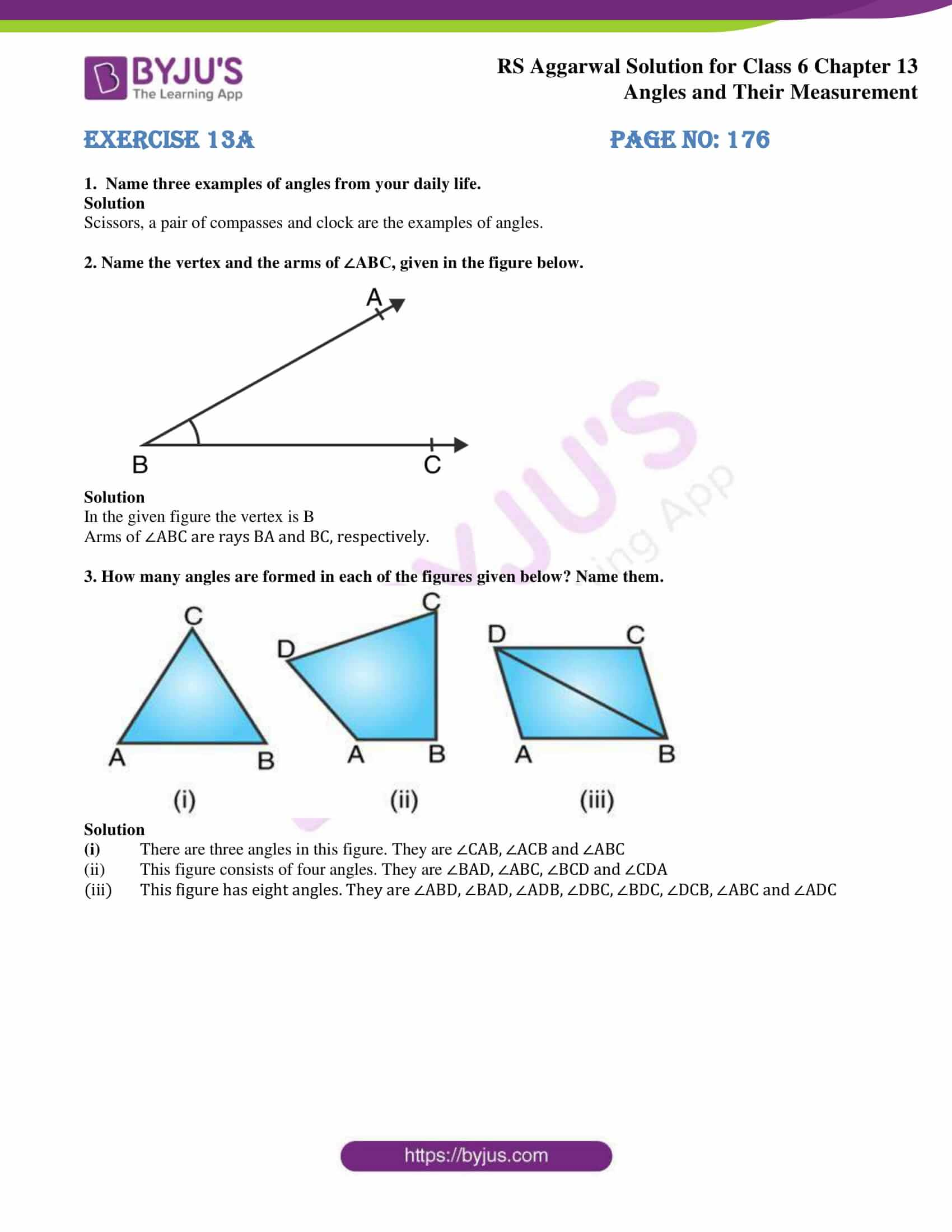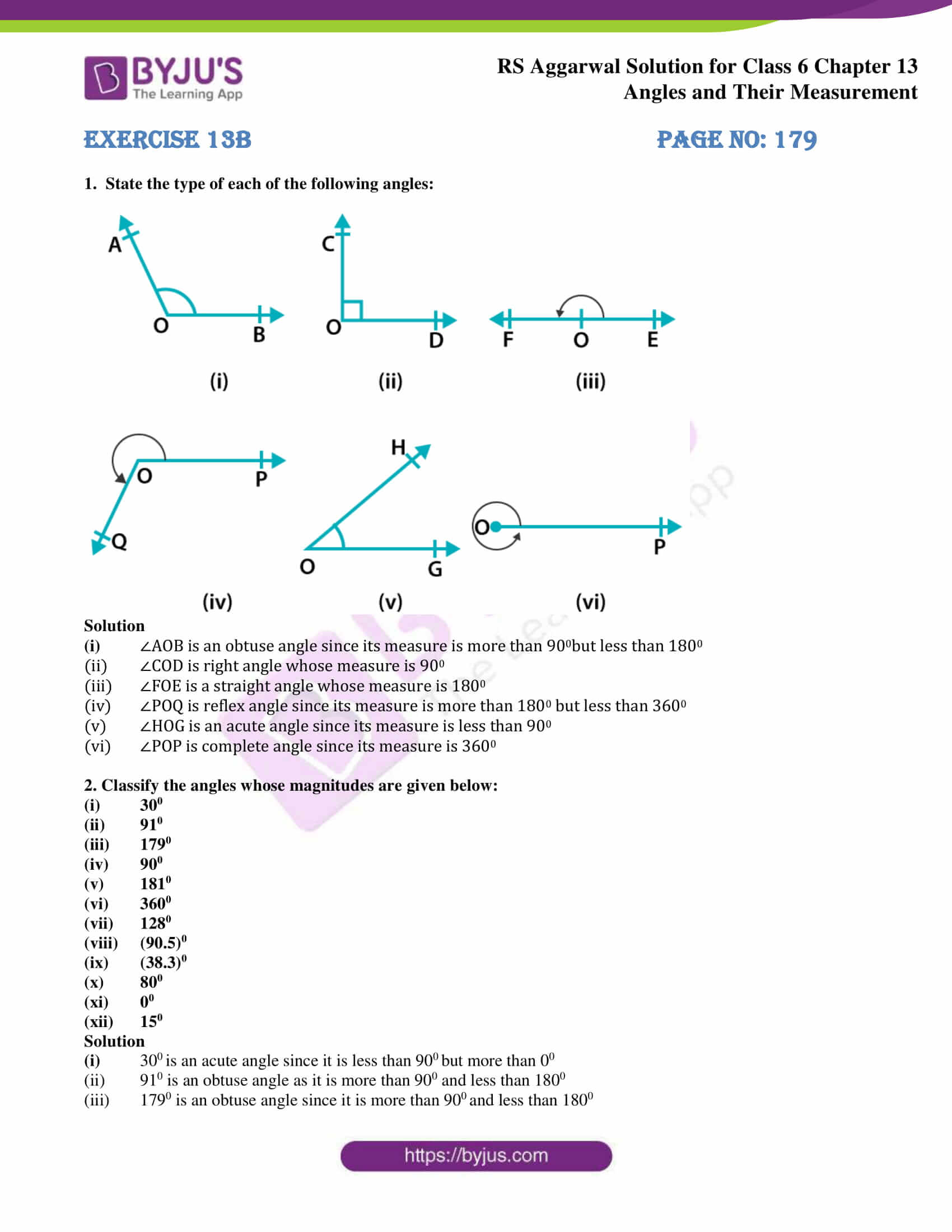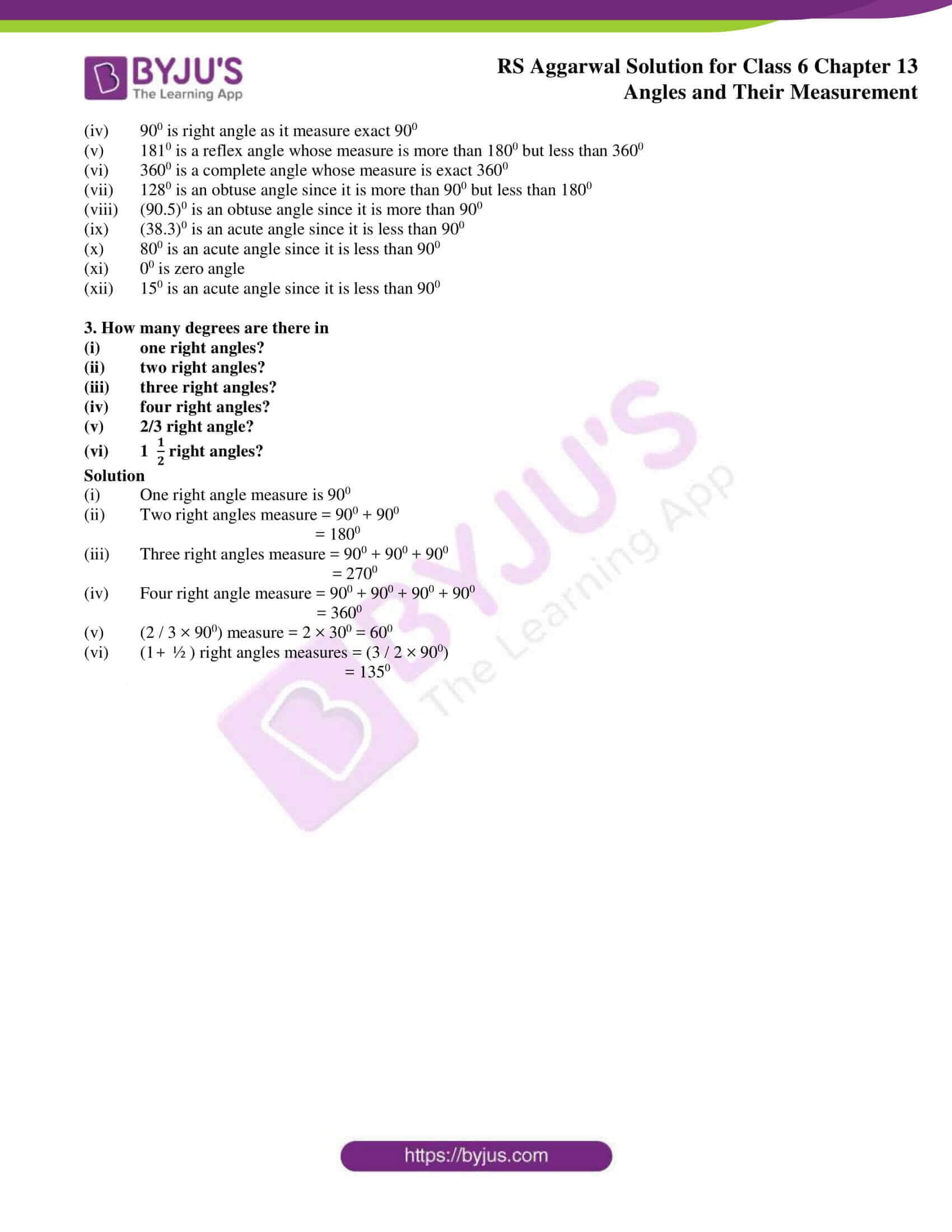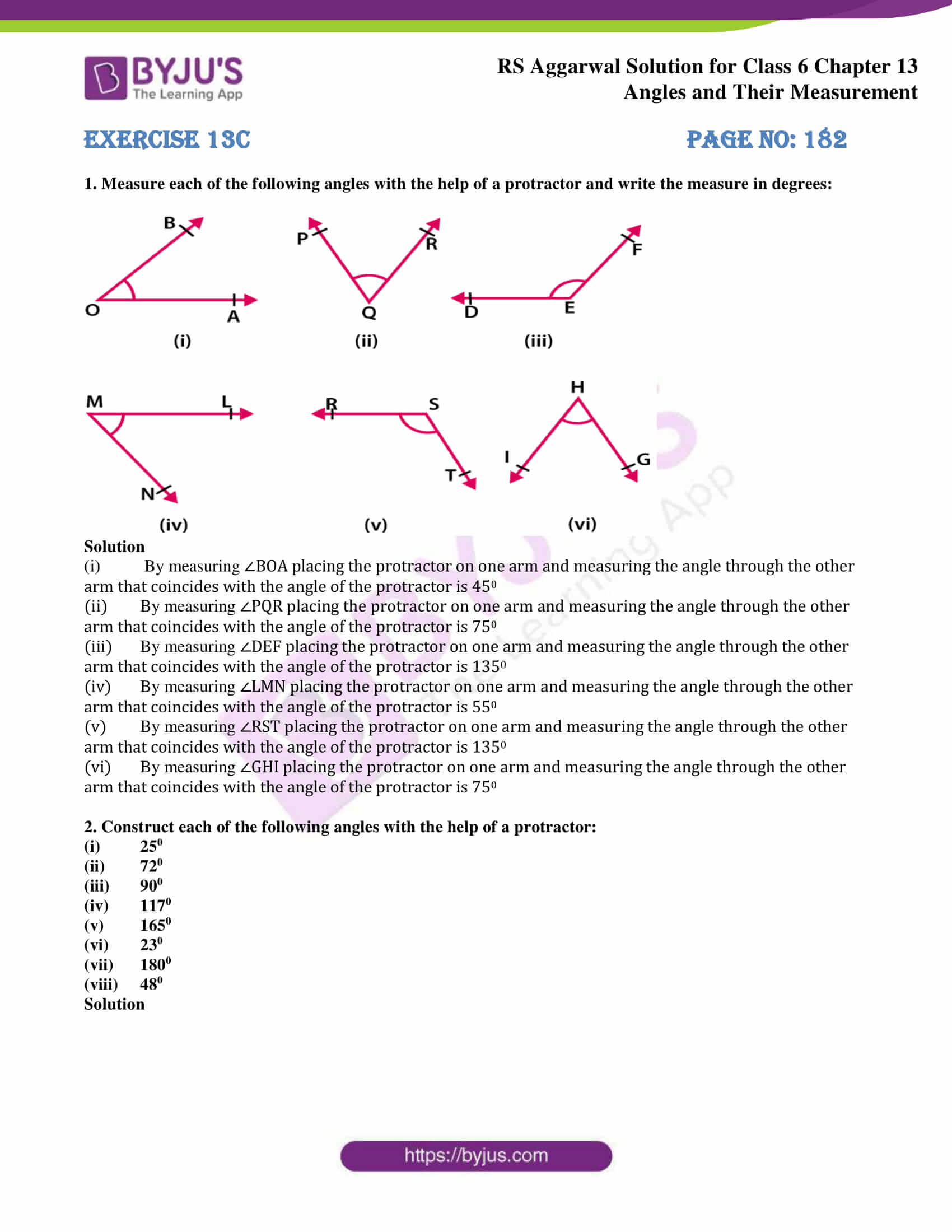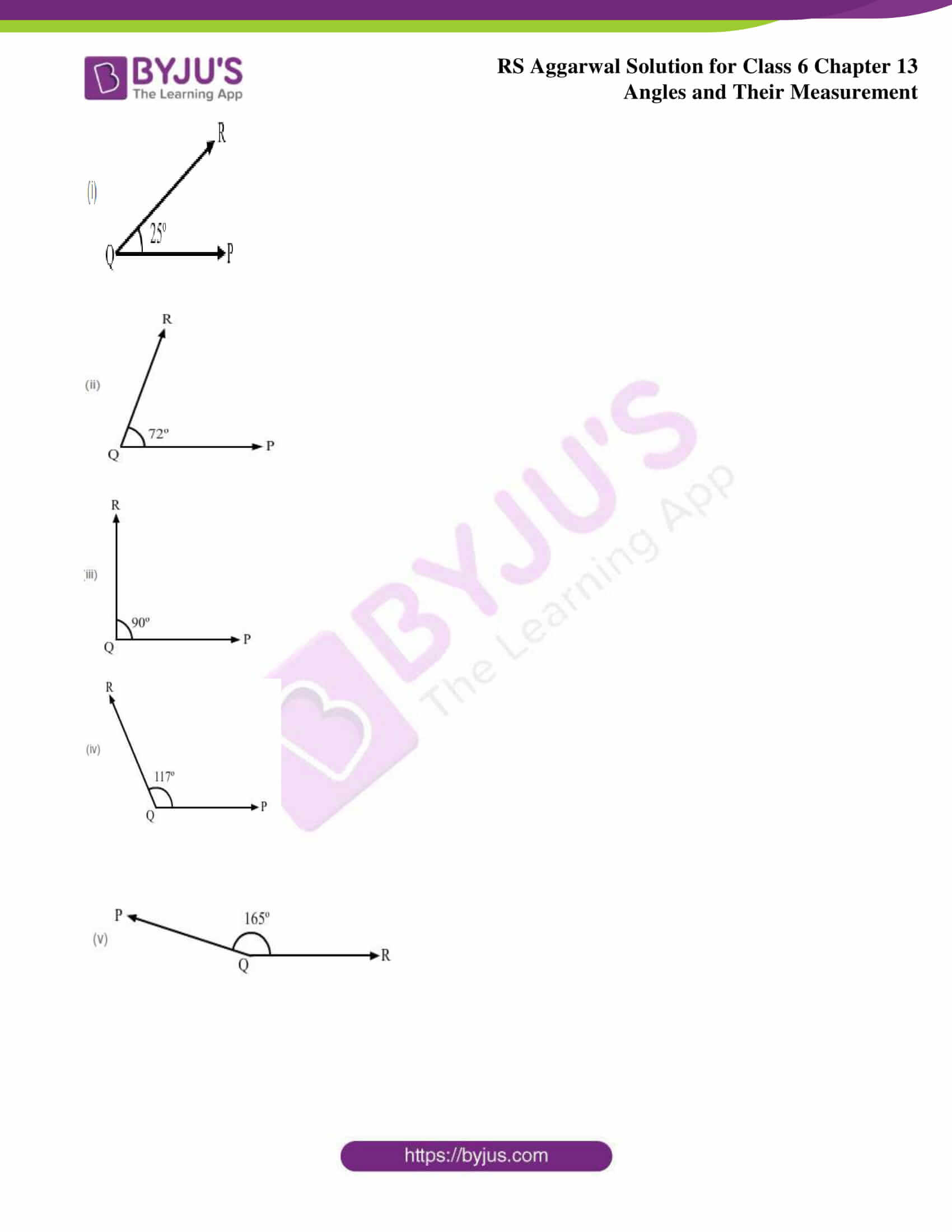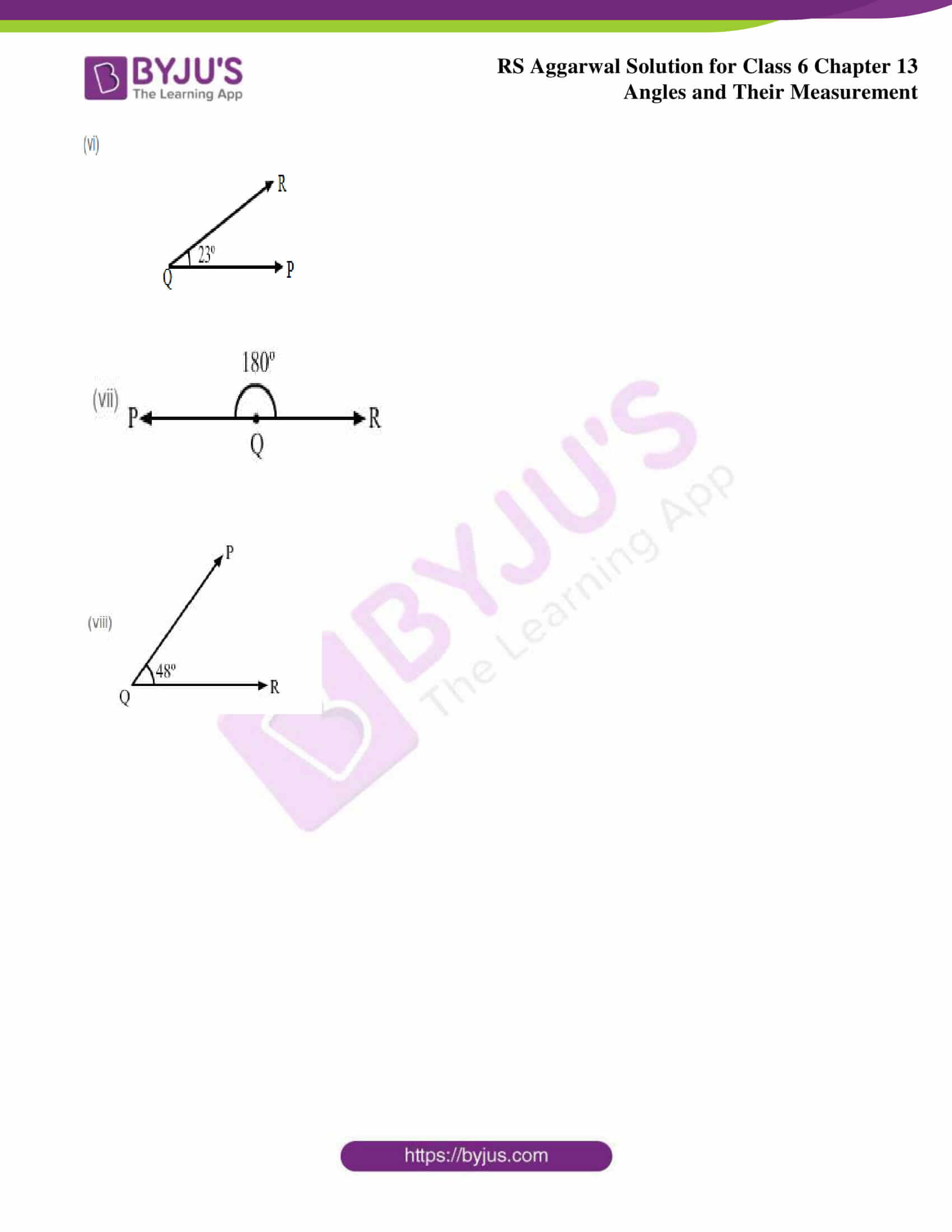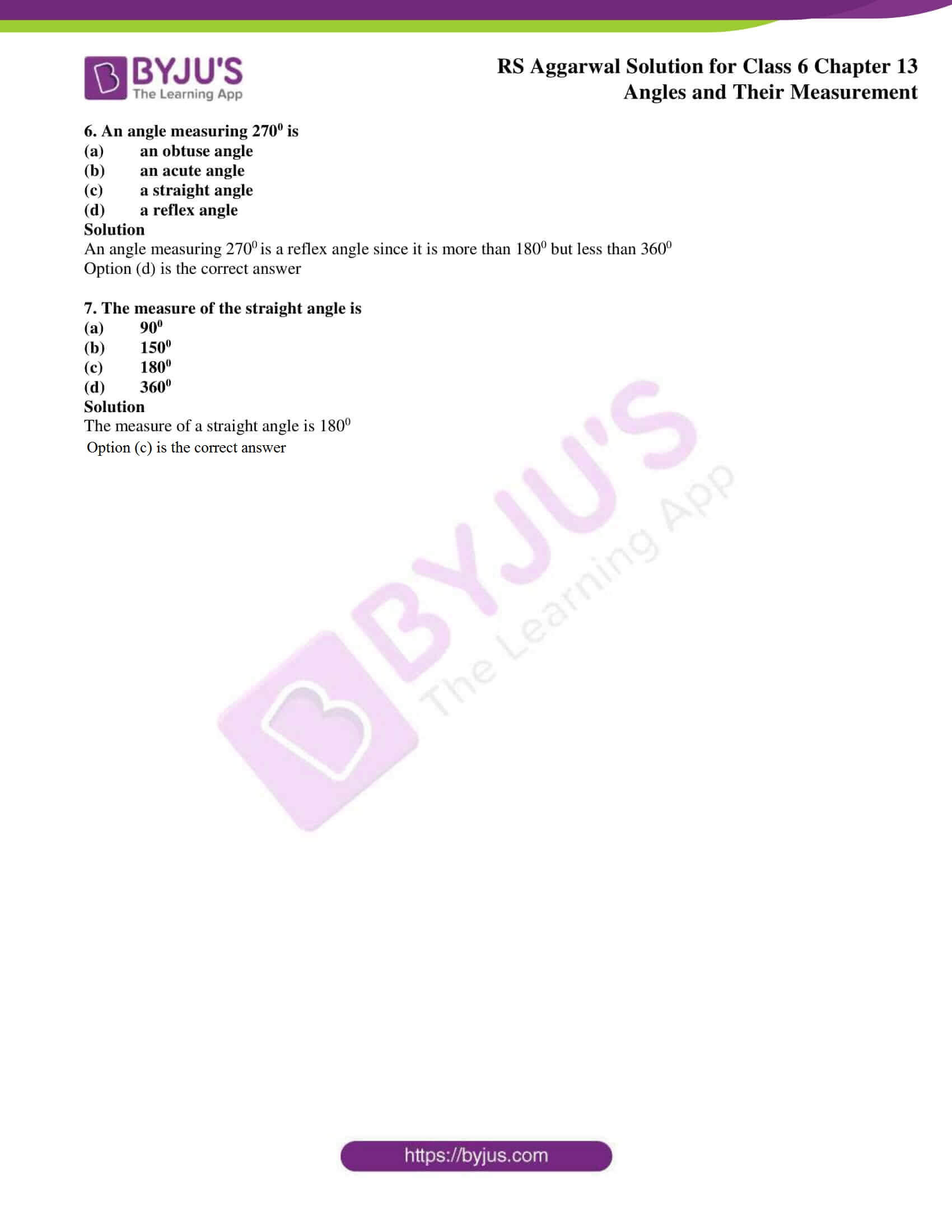### Access answers of RS Aggarwal Solutions for Class 6 Maths Chapter 13 – Angles and their Measurement

Exercise 13A PAGE NO: 176

1. Name three examples of angles from your daily life.

Solution

Scissors, a pair of compasses and clock are the examples of angles.

2. Name the vertex and the arms of ∠ABC, given in the figure below.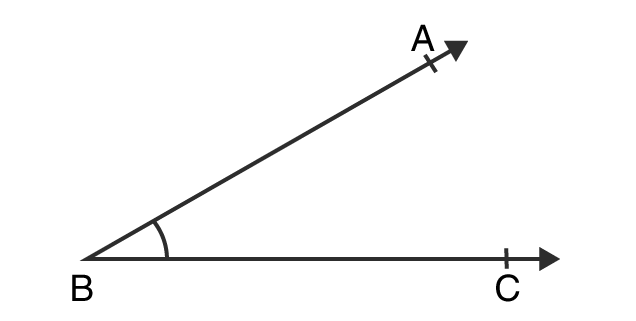Solution

In the given figure the vertex is B

Arms of ∠ABC are rays BA and BC, respectively.

3. How many angles are formed in each of the figures given below? Name them.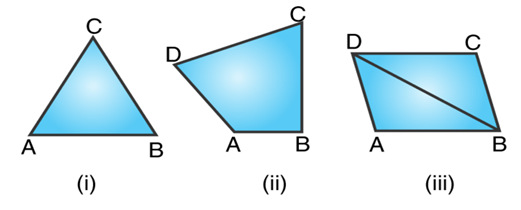Solution

(i) There are three angles in this figure. They are ∠CAB, ∠ACB and ∠ABC

(ii) This figure consists of four angles. They are ∠BAD, ∠ABC, ∠BCD and ∠CDA

(iii) This figure has eight angles. They are ∠ABD, ∠BAD, ∠ADB, ∠DBC, ∠BDC, ∠DCB, ∠ABC and ∠ADC

Exercise 13B PAGE NO: 179

1. State the type of each of the following angles: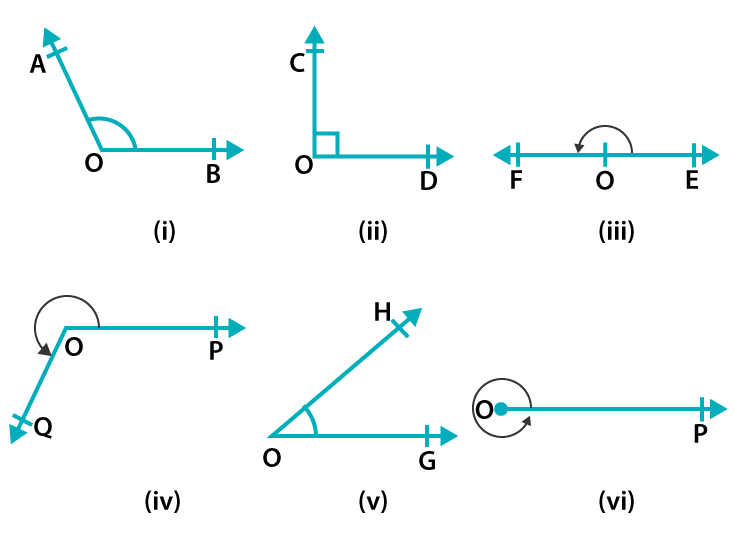Solution

(i) ∠AOB is an obtuse angle since its measure is more than 900but less than 1800

(ii) ∠COD is right angle whose measure is 900

(iii) ∠FOE is a straight angle whose measure is 1800

(iv) ∠POQ is reflex angle since its measure is more than 1800 but less than 3600

(v) ∠HOG is an acute angle since its measure is less than 900

(vi) ∠POP is complete angle since its measure is 3600

2. Classify the angles whose magnitudes are given below:

(i) 300

(ii) 910

(iii) 1790

(iv) 900

(v) 1810

(vi) 3600

(vii) 1280

(viii) (90.5)0

(ix) (38.3)0

(x) 800

(xi) 00

(xii) 150

Solution

(i) 300 is an acute angle since it is less than 900 but more than 00

(ii) 910 is an obtuse angle as it is more than 900 and less than 1800

(iii) 1790 is an obtuse angle since it is more than 900 and less than 1800

(iv) 900 is right angle as it measure exact 900

(v) 1810 is a reflex angle whose measure is more than 1800 but less than 3600

(vi) 3600 is a complete angle whose measure is exact 3600

(vii) 1280 is an obtuse angle since it is more than 900 but less than 1800

(viii) (90.5)0 is an obtuse angle since it is more than 900

(ix) (38.3)0 is an acute angle since it is less than 900

(x) 800 is an acute angle since it is less than 900

(xi) 00 is zero angle

(xii) 150 is an acute angle since it is less than 900

3. How many degrees are there in

(i) one right angles?

(ii) two right angles?

(iii) three right angles?

(iv) four right angles?

(v) 2/3 right angle?

(vi) 1 (1/2) right angles?

Solution

(i) One right angle measure is 900

(ii) Two right angles measure = 900 + 900

= 1800

(iii) Three right angles measure = 900 + 900 + 900

= 2700

(iv) Four right angle measure = 900 + 900 + 900 + 900

= 3600

(v) (2 / 3 × 900) measure = 2 × 300 = 600

(vi) (1 + ½ ) right angles measures = (3 / 2 × 900)

= 1350

Exercise 13C PAGE no: 182

1. Measure each of the following angles with the help of a protractor and write the measure in degrees: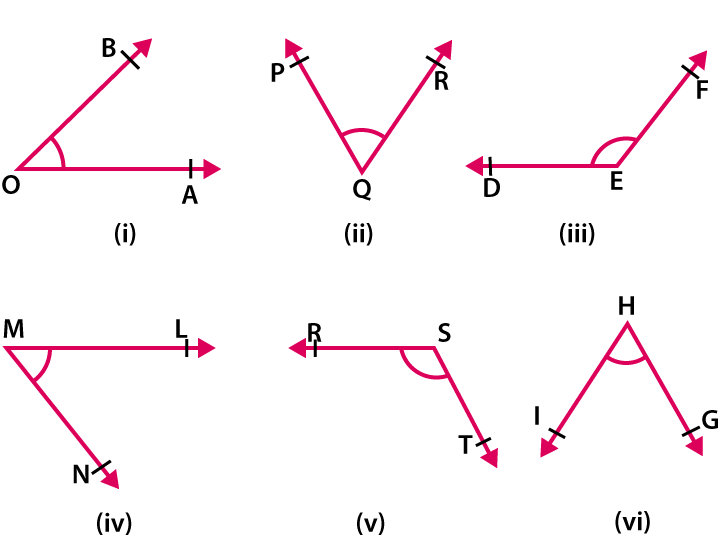Solution

(i) By measuring ∠BOA placing the protractor on one arm and measuring the angle through the other arm that coincides with the angle of the protractor is 450

(ii) By measuring ∠PQR placing the protractor on one arm and measuring the angle through the other arm that coincides with the angle of the protractor is 750

(iii) By measuring ∠DEF placing the protractor on one arm and measuring the angle through the other arm that coincides with the angle of the protractor is 1350

(iv) By measuring ∠LMN placing the protractor on one arm and measuring the angle through the other arm that coincides with the angle of the protractor is 550

(v) By measuring ∠RST placing the protractor on one arm and measuring the angle through the other arm that coincides with the angle of the protractor is 1350

(vi) By measuring ∠GHI placing the protractor on one arm and measuring the angle through the other arm that coincides with the angle of the protractor is 750

2. Construct each of the following angles with the help of a protractor:

(i) 250

(ii) 720

(iii) 900

(iv) 1170

(v) 1650

(vi) 230

(vii) 1800

(viii) 480

Solution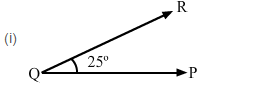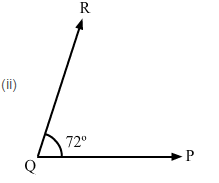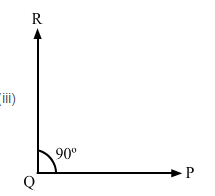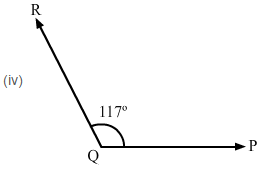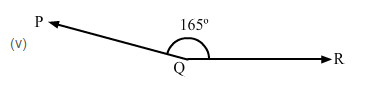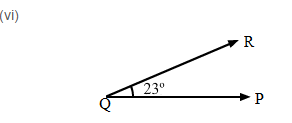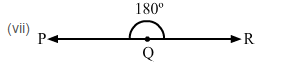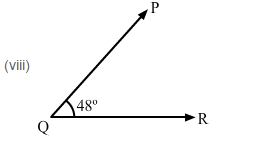Exercise 13D PAGE NO: 182

Objective Questions

Mark () against the correct answer in each of the following:

1. Where does the vertex of an angle lie?

(a) In its interior

(b) In its exterior

(c) On the angle

(d) None of these

Solution

The common initial point is known as vertex of the angle. Hence, it lies on the angle

Option (c) is the correct answer

2. The figure formed by two rays with the same initial point is called

(a) a ray

(b) a line

(c) an angle

(d) none of these

Solution

The figure formed by the angle with the same initial point is called an angle

Option (c) is the correct answer

3. An angle measuring 1800 is called

(a) a complete angle

(b) a reflex angle

(c) a straight angle

(d) none of this

Solution

An angle measuring 1800 is a straight line

Option (c) is the correct answer

4. An angle measuring 900 is called

(a) a straight angle

(b) a right angle

(c) a complete angle

(d) a reflex angle

Solution

An angle measuring 900 is called a right angle

Option (b) is the correct answer

5. An angle measuring 910 is

(a) an acute angle

(b) an obtuse angle

(c) a reflex angle

(d) none of this

Solution

An angle measuring 910 is called an obtuse angle which is more than 900 but less than 1800

Option (b) is the correct answer

6. An angle measuring 2700 is

(a) an obtuse angle

(b) an acute angle

(c) a straight angle

(d) a reflex angle

Solution

An angle measuring 2700 is a reflex angle since it is more than 1800 but less than 3600

Option (d) is the correct answer

7. The measure of the straight angle is

(a) 900

(b) 1500

(c) 1800

(d) 3600

Solution

The measure of a straight angle is 1800

Option (c) is the correct answer

### RS Aggarwal Solutions for Class 6 Maths Chapter 13 Angles and their Measurement

Chapter 13 – Angles and their Measurement consists of four exercises. Each question of every exercise present in RS Aggarwal Solutions are well explained. Let’s have a look at the topics which are talked about in this chapter.

• Magnitude of an angle and its measurement
• Measuring an angle by a protractor
• Perpendicular Lines

Also, access RS Aggarwal Solutions for Class 6 Chapter 13 Exercises

Exercise 13A

Exercise 13B

Exercise 13C

Exercise 13D

### Chapter Brief of RS Aggarwal Solutions for Class 6 Maths Chapter 13 Angles and their Measurement

The figure formed by two rays with the same initial point is called an angle. The common initial point is known as vertex of the angle, and the rays forming the angle are called its arms or sides. Types of angles are acute angle, obtuse angle, right angle, straight angle, reflex angle, complete angle and zero angle. Clock, compass, arms are examples of angles.